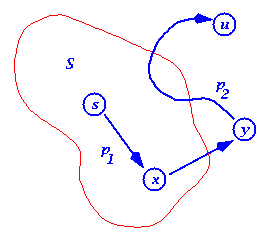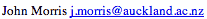Data Structures and Algorithms Proof of Dijkstra's Algorithm

We use a proof by contradiction again. But first, we assert the following Lemma:

 Lemma 1 Shortest paths are composed of shortest paths. The proof of this is based on the notion that if there was a shorter path than any sub-path, then the shorter path should replace that sub-path to make the whole path shorter. Lemma 2 If s ->..-> u -> v is a shortest path from s to v, then after u has been added to S and relax(u,v,w) called, then d[v] = delta(s,v) and d[v] is not changed thereafter. For formal proofs, see Cormen or any one of the texts which cover this important algorithm. Proof follows from the fact that at all times d[v] >= delta(s,v).

Denote the distance of the shortest path from s to u as delta(s,u). After running Dijkstra's algorithm, we assert that d[u] = delta(s,u) for all u.

Note that once u is added to S, d[u] is not changed and should be delta(s,u).

Suppose that u is the first vertex added to S for which d[u] != delta(s,u). We note:

1. u cannot be s, because d[s] = 0.
2. There must be a path from s to u. If there were not, d[u] would be infinity.
3. Since there is a path, there must be a shortest path.Let s -(p1)-> x -> y -(p2)-> u be the shortest path from s to u. Where x is within S and y is the first vertex not within S.

When x was inserted into S, d[x] = delta(s,x) (since we hypothesise that u was the first vertex for which this was not true).

Edge (x,y) was relaxed at that time, so that
d[y] = delta(s,y)
<= delta(s,u)
<= d[u]

Now both y and u were in V-S when u was chosen, so d[u] <= d[y].

Thus the two inequalities must be equalities,
d[y] = delta(s,y) = delta(s,u) = d[u]

So d[u] = delta(s,u) contradicting our hypothesis.

Thus when each u was inserted, d[u] = delta(s,u).

QED

©, 1998